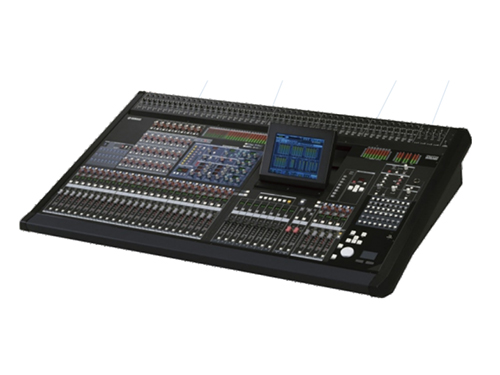###### 广东三毛演出177 2774 17774新闻动态

# 迷宫音响的注意事项有哪些你知道吗

﻿如果设计的迷宫式音箱的喇叭单元基本谐振峰与声管的反谐振点相同，低频的下潜能力就会降低至1.33倍。迷宫式音箱的设计，一般是先测出所采用的喇叭单元本身的谐振频率fo，用下式求得放音管道的长度：声管长度=n×344/4×fo……（1），式中n是奇数值的1、3、5、7，344是声波的速度，将不同的奇数值的n代入公式，看哪个值与设计的低频下限相近，即是最佳的n数值。迷宫式音箱的重放下限频率可按下式求得：f放=2×fo/n……（2）例如手边现有一对8喇叭单元，欲设计一款迷宫式音箱，这只8寸单元fo=35Hz用公式
（1）求放音管道的长度：
1.n=1时，声管长度（米）=1×344/4×35=344/140=2.45m
2.n=3时，声管长度（米）=3×344/4×35=1032/140=7.37m
3.n=5时，声管长度（米）=5×344/4×35=1720/140=12.28m

1.n=1时，f放=2×35/n=70/1=70Hz
2.n=3时，f放=2×35/3=70/3=23.3Hz
3.n=5时，f放=2×35/5=70/5=14Hz177-2774-17772546611948@qq.com